Latest Banking jobs   »   Reasoning Ability Quiz For IBPS PO...

# Reasoning Ability Quiz For IBPS PO Prelims 2021- 26th November

Directions (1-2): Read the following information carefully and answer the question which follows:
IF ‘A × B’ means ‘A is son of B’
IF ‘A + B’ means ‘A is daughter of B’
IF ‘A ÷ B’ means ‘A is wife of B’
IF ‘A – B’ means ‘A is Father of B’

Q1. What will come in the place of the question mark, to establish that M is mother of J in the expression?
‘J + K – L ? M’
(a) +
(b) ×
(c) –
(d) ÷
(e) Either (a) or (b)

Q2. Which of the following relations are true based upon the relations given in the question?
J ÷ K × L – M + N’?
(a) K is brother of N
(b) J is daughter-in-law of M
(c) J is daughter-in-law of N
(d) N is daughter of L
(e) None is true

Directions (3-4): Read the following information carefully and answer the questions given below:
There are seven members S, T, U, V, W, X and Y in a family among them only 3 females. S is married to V. W is grandson of V who has two children. U is sister in law of Y who is unmarried. X is brother in law of S who is mother in law of U.

Q3. How X is related to Y?
(a) Uncle
(b) Brother
(c) Mother
(d) Sister
(e) Grandson

Q4. Who among the following is the father of W?
(a) U
(b) T
(c) X
(d) Y
(e) None of these

Directions :(5-7) Study the following information carefully and answer the questions which follow–

‘P – Q’ means ‘P is father of Q’
‘P ÷ Q’ means ‘P is sister of Q’
‘P × Q’ means ‘P is mother of Q’
‘P + Q’ means ‘P is brother of Q’

Q5. Which of the following means ‘A is nephew of B’?
(a) A + C – B × K
(b) B ÷ H – A + D
(c) B ÷ G – A ÷ R
(d) B + T × A ÷ E
(e) None of these

Q6. Which of the following means ‘P is grandfather of J’?
(a) J ÷ W – U – P
(b) P × G + J ÷ A
(c) P – B ÷ J ÷ R
(d) P – T – J ÷ S
(e) None of these

Q7. How is R related to B in the expression ‘B ÷ C – S + R’?
(a) aunt
(b) niece
(c) nephew
(d) None of these
(e) Cannot be determined

Directions (8-9): Study the information carefully and answer the questions given below.
P is father of M who is brother in law of K. N is daughter in law of Z who is grandmother of L. P has only two children one son and one daughter. X is sister in law of N. P is father in law of N. K is unmarried.

Q8. If K is wife of J then what could be the relation of J with respect to N?
(a) Brother
(b) Brother in law
(c) Uncle
(d) Cannot be determined
(e) None of these.

Q9. What is relation of L with respect to X?
(a) Nephew
(b) Niece
(c) Son
(d) Daughter
(e) Cannot be determined

Directions (10-11): Study the following information carefully to answer the given questions
P × Q means P is father of Q
P ÷ Q means P is daughter of Q
P + Q means P is sister of Q
P – Q means P is husband of Q

Q10. In M ÷ N × O – P, How O related to M?
(a)Brother
(b)Son –in-law
(c)Father
(d)Son
(e)None of these

Q11. In G×T+Q÷M, how is M related to G?
(a)Mother
(b)Sister
(c)Wife
(d)Daughter
(e)None of these.

Directions (12-13): Study the information carefully and answer the questions given below.

M, N, O, P, Q and R are six family members. Q is son of P, who is not the mother of Q. N is brother of P. R and P are a married couple. O is daughter of R, who is sister of M.

Q12. How many female members are there in the family?
(a) Two
(b) Three
(c) Four
(d) Can’t be determined
(e) None of these

Q13. How is Q related to O?
(a) Mother
(b) Father
(c) Sister
(d) Brother
(e) None of these

Directions :(14-15) Study the following information carefully and answer the questions which follow–
‘P – Q’ means ‘P is father of Q’
‘P ÷ Q’ means ‘P is sister of Q’
‘P × Q’ means ‘P is mother of Q’
‘P + Q’ means ‘P is brother of Q’

Q14. Which of the following means ‘A is nephew of B’?
(a) A + C – B × K
(b) B ÷ H – A + D
(c) B ÷ G – A ÷ R
(d) B + T × A ÷ E
(e) None of these

Q15. Which of the following means ‘P is grandfather of J’?
(a) J ÷ W – U – P
(b) P × G + J ÷ A
(c) P – B ÷ J ÷ R
(d) P – T – J ÷ S
(e) None of these

Solutions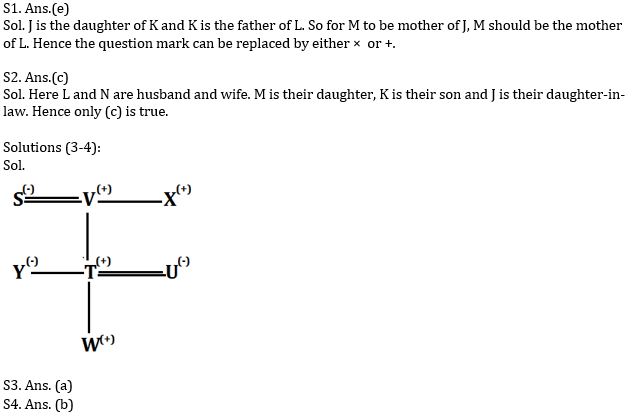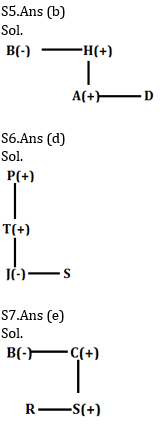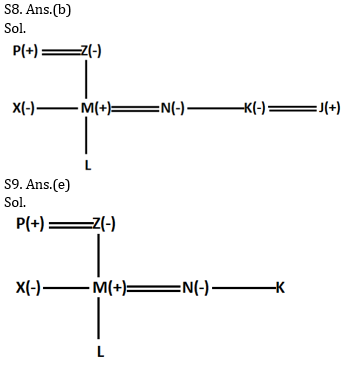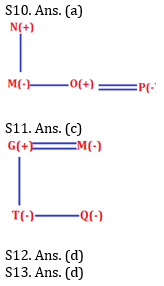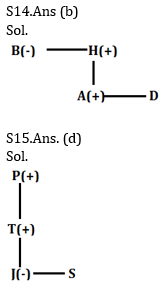×

Thank You, Your details have been submitted we will get back to you.Join India's largest learning destination

What You Will get ?

•Job Alerts
•Daily Quizzes
•Subject-Wise Quizzes
•Current Affairs
•Previous year question papers
•Doubt Solving session

ORJoin India's largest learning destination

What You Will get ?

•Job Alerts
•Daily Quizzes
•Subject-Wise Quizzes
•Current Affairs
•Previous year question papers
•Doubt Solving session

ORJoin India's largest learning destination

What You Will get ?

•Job Alerts
•Daily Quizzes
•Subject-Wise Quizzes
•Current Affairs
•Previous year question papers
•Doubt Solving session

Enter the email address associated with your account, and we'll email you an OTP to verify it's you.Join India's largest learning destination

What You Will get ?

•Job Alerts
•Daily Quizzes
•Subject-Wise Quizzes
•Current Affairs
•Previous year question papers
•Doubt Solving session

Enter OTP

Please enter the OTP sent to
/6

Did not recive OTP?

Resend in 60sJoin India's largest learning destination

What You Will get ?

•Job Alerts
•Daily Quizzes
•Subject-Wise Quizzes
•Current Affairs
•Previous year question papers
•Doubt Solving sessionJoin India's largest learning destination

What You Will get ?

•Job Alerts
•Daily Quizzes
•Subject-Wise Quizzes
•Current Affairs
•Previous year question papers
•Doubt Solving session

Almost there

+91Join India's largest learning destination

What You Will get ?

•Job Alerts
•Daily Quizzes
•Subject-Wise Quizzes
•Current Affairs
•Previous year question papers
•Doubt Solving session

Enter OTP

Please enter the OTP sent to Edit Number

Did not recive OTP?

Resend 60

By skipping this step you will not recieve any free content avalaible on adda247, also you will miss onto notification and job alerts

Are you sure you want to skip this step?

By skipping this step you will not recieve any free content avalaible on adda247, also you will miss onto notification and job alerts

Are you sure you want to skip this step?